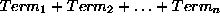Next: The fd Symbolic Finite Up: Solvers and Syntax Previous: Solvers and Syntax

## The fd (Finite Domain) Library

The finite domain library has been used and refined over a 10 year period. As a result it has a great many constraint handling facilities. It is best seen as three libraries.

The first is a library for handling symbolic finite domains, with values like red, machine_1 etc. The built-in constraints on symbolic finite domain variables are equations and disequalities: these constraints can only hold between expressions which are either constants or variables. These constraints can also be used when the domains are numeric.

The second is a library for handling integer variables, and numerical constraints on those variables. The library propagates equations and inequalities between linear expressions. A linear numeric expression is one that can be written in the form, where each term can, in turn, be written Number or Number * Variable. The number can be positive or negative. An example is the expression 3*X + (-4)*Y + 3 (which we would normally write 3*X - 4*Y + 3).

The third is a library supporting some built-in complex constraints. Two examples of such constraints are the `alldistinct` constraint, which constraints a set of variables to take values which are pairwise distinct, and the `atmost` constraint, which constrains at most N variables from a given set to take a certain value.

Joachim Schimpf
Wed Sep 3 18:07:19 BST 1997